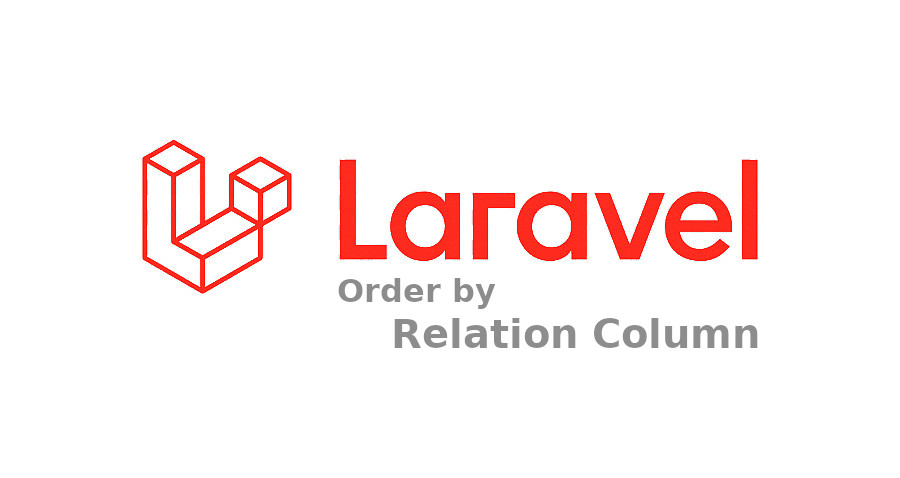# Laravel order by relation column with example

Some examples that demonstrate the usage of Laravel’s orderBy method with relationship columns, which can be applied in Laravel versions 5, 6, 7, 8, 9, and 10.In this post, we focus on examples to use Laravel order by relation column. There are many different ways to achieve it.

Below are some examples that demonstrate the usage of Laravel‘s orderBy method with relationship columns. These examples can be applied in Laravel versions 5, 6, 7, 8, 9, and 10.

## Example 1: Ordering by a BelongsTo Relationship Column

Consider the scenario where you have a `"User"` model that belongs to a `"Role"` model. You can use the `orderBy` method to sort the users based on the role name in ascending order.

``````\$users = User::with(['role' => function (\$q) {
\$q->orderBy('name');
}])->get();``````

You can use the same code for descending order by adding `'desc'` argument to `orderBy` method as follows,

``````\$users = User::with(['role' => function (\$q) {
\$q->orderBy('name', 'desc');
}])->get();``````

For above example to work properly, you have to define `belongsTo` relationship of `"Role"` model inside the `"User"` model as follows,

``````public function role(): BelongsTo
{
return \$this->belongsTo(Role::class, 'role_id', 'id');
}``````

## Example 2: Using inner join with relation table

For above scenario, where “User” model that belongs to “Role” model, you can use join method to perform user sorting based on role name as follows,

``````\$users = User::select('*')
->join('roles', 'users.role_id', '=', 'roles.id')
->orderBy('roles.name', 'asc');``````

As above example, you need to replace the second argument of `orderBy` method to `'desc'` for sort records in descending order. There is no need of any relationship required for this query.

## Example 3: Using sortBy() and sortByDesc() methods

You can use sortBy() and sortByDesc() methods to order the records for the same scenario as follows,

``````\$users = User::get()->sortBy(function(\$query){
return \$query->role->name;
})->all();``````

In above example, it first get the users collection from the database and then sort them by the provided relation column. For these methods, you have to define `belongsTo` relationship in `"User"` model.

You can use sortByDesc() method same as above.

## Example 4: Using subquery and whereColumn method

You can also use subqueries to sort records. For the above scenario, you can use subqueries as follows,

``````\$users = User::select('*')
->orderBy(Role::select('name')
->whereColumn('roles.id', 'users.role_id')
);``````

In above example, we have we used subquery from `roles` table using `whereColumn` method to get the name of the role of each row of `users` table. After getting the role name, we used `orderBy` method to achieve the sorted records.

For sorting records in descending order, you can use the above example with `orderByDesc` method.

You can use this example, without defining `belongsTo` relationship in model.

These are some useful examples to order records based on relationship model in Laravel.